Confidence Intervals

### Parameter Confidence Intervals

The parameter confidence intervals are computed using the Wald-based formula: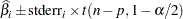whereis theth parameter estimate,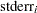is its estimated approximate standard error,is astatistic withdegrees of freedom,is the number of observations, andis the number of parameters. The confidence intervals are only asymptotically valid. The significance levelused in the construction of these confidence limits can be set with the ALPHA= option in the PROC NLIN statement; the default value is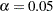.

### Model Confidence Intervals

Model confidence intervals are output when an OUT= data set is specified and one or more of the keywords LCLM, UCLM, LCL, UCL, L95M=, U95M=, L95=, and U95= is specified. The expressions for these terms are as follows: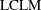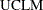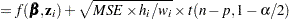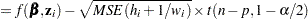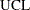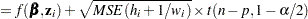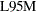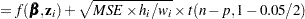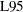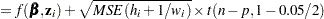whereis the leverage,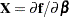, and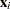is theth row of. These results are derived for linear systems. The intervals are approximate for nonlinear models. The valuein the preceding formulas for LCLM, UCLM, LCL, and UCL can be set with the ALPHA= option in the PROC NLIN statement or with the ALPHA= option in the OUTPUT statement. If both ALPHA= options are specified, the option in the OUTPUT takes precedence.